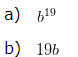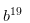# Algebraic Expressions for Class 6 Worksheets

Please check Solutions of Algebraic Expressions for Class 6 Worksheets at the end of the questions.

Download Algebraic Expressions for Class 6 Worksheets1. Identify the numerical coefficient of terms (other than constant) in the given expression.
4x+3y+7
a) 4, 3, 7
b) 4, 3

2. State whether a given pair of terms is of Like terms or Unlike terms?
7x, 7y
a) Like terms
b) Unlike terms

3. State whether a given pair of terms is of Like terms or Unlike terms?
4xy, 7xy
a) Like terms
b) Unlike terms

4. Write the following in exponential form:
​​b x b x b x b x b x 19 times5. If a = 7 and b = 3 then, Find the value of a + 6b
a) 25
b) 39

6. Check whether the given equation is true or false on substituting the value of variables?
5a – 2b = 3c , when a = 3 , b = 4 and c = 2
a) True
b) False

7. Subtract 5a from 13a.
a) 6a
b) 4a
c) 8a

8. Add the terms 7a and 4a
a) 11a
b) 11a²

### Algebraic Expressions for Class 6 Worksheets Solutions

Algebraic Expressions for Class 6 Worksheets – Solution 1

4x+3y+7
In the given expression, various terms (other than the constant 7 ) are as under : –
4x – Numerical Coefficient of 4x is 4.
3y – Numerical Coefficient of 3y is 3.

Correct Answer – b) 4, 3

Algebraic Expressions for Class 6 Worksheets – Solution 2

When two terms have same variables and same power of each variable then those terms are called like terms otherwise unlike terms. The constant can be different. All constants are like terms.

The given terms are Unlike Terms, since both the terms have different variables , i.e, the first term 7x has a variable x and the second term 7y has a variable y.

Correct Answer – b) Unlike terms

Solution 3

When two terms have same variables and same power of each variable then those terms are called like terms otherwise unlike terms. The constant can be different. All constants are like terms.

Both the given terms are Like Terms, since both the terms have same variables , i.e, “xy”

Correct Answer – a) Like terms

Solution 4

​Given
b x b x b x b x b x 19 times
= b x 19 times
=Correct Answer – a) ​Algebraic Expressions for Class 6 Worksheets – Solution 5

Given:
a = 7
b = 3
Given expression
a + 6b
Now we will replace a = 7 and b = 3
= 7 + 6 x 3
According to BODMAS rule we will do multiplication first
= 7 + 18
then, Addition
= 25

Correct Answer – a) 25

Algebraic Expressions for Class 6 Worksheets – Solution 6

LHS: 5a – 2b
Substitute the value, a = 3 and b = 4 in the given expression
We get,
( 5 x a ) – ( 2 x b )
= ( 5 x 3 ) – ( 2 x 4 )
According to BODMAS rule we will do multiplication first
= 15 – 8
then, Addition
= 7
On Solving RHS,
RHS = 3c
Substituting the value , c = 2
RHS = 3 x 2
RHS = 6
Hence, LHS ≠ RHS
So, the above expression is FALSE

Correct Answer – b) False

Algebraic Expressions for Class 6 Worksheets – Solution 7

Given Expression :
13a and 5a
Both the expression have same Variable so we can substract them,
Here,
Constants = 13 and 5
Variable = a
Therefore,
13a – 5a
Taking ” a ” as common we get
= ( 13 – 5 )a
= 8a

Correct Answer – c) 8a

Algebraic Expressions for Class 6 Worksheets – Solution 8

Since both the expression have same Variable, we can add them
Given expression are 7a and 4a
Here 7 and 4 are constants
and ” a ” is variable
7 x a + 4 x a
Taking “a” as common
( 7 + 4 )a
11a

Correct Answer – a) 11a